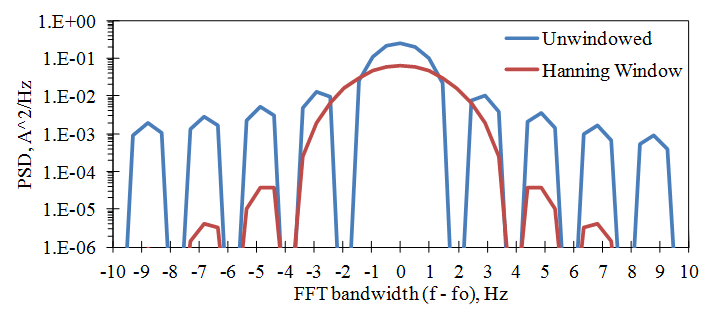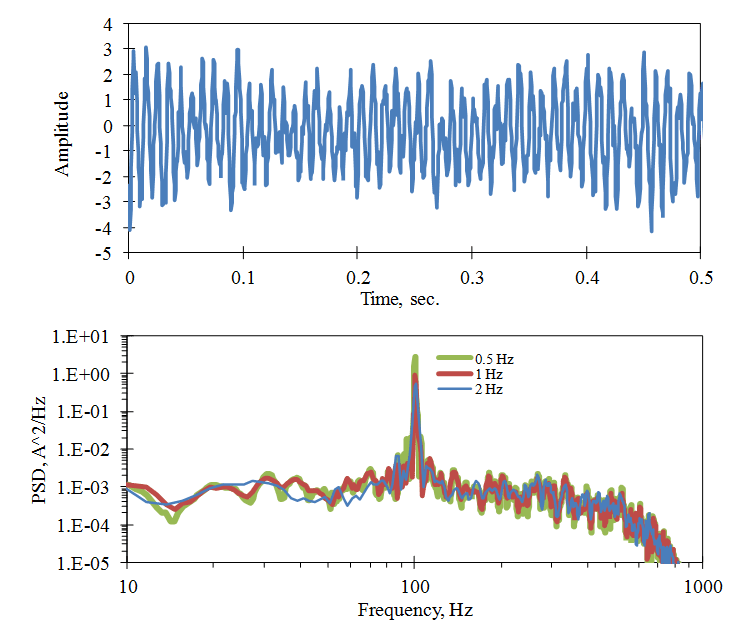# Frequency Resolution

March 29, 2018

The basic frequency resolution in the PSD is the frequency spacing of the FFT, Δf = 1 / T, where T is the length of the time sample. However, it is important to understand how the effective frequency resolution is changed by the windowing function used and how the frequency resolution affects the evaluation of resonance peaks.

In the lesson on Windowing, it is shown that sideband leakage occurs when a window function is applied to the digitized signal. The benefit of applying a window before computing the FFT is that it reduces the remote band noise generated by non-periodic components in the signal. The trade-off is that the effective width of each FFT frequency band is increased. This is because the windowing process slightly distorts the signal and makes the frequency resolution of the FFT less precise. This can be seen by comparing the PSD of the periodic signal with and without windowing as used in Figures 2.19 and 2.21.  Figure 2.22 compares these frequency peaks using 4 times the frequency resolution of the base FFT and plots the frequency axis relative to the frequency of the sine wave, fo.Figure 2.22. Comparison of the FFT bandwidth with and without windowing. (Base FFT using SR = 2000 Hz, N = 1024, Δf = 1.953 Hz.)

For the case without a window (sometimes called a “rectangular window”), the frequency resolution is about ± Δf /2 for this case (ignoring the remote band leakage). With the Hanning window applied, the frequency resolution spreads out to about ± 3 Δf /2. That means pure tones can only be resolved to accuracy with a range three times that of the base FFT resolution. Another measure of this is the equivalent noise bandwidth which measures the relative mean square amplitude in a frequency band when the signal is white noise. This is determined by the half-power bandwidth, which is based on the frequencies where the band level is 1/2 the peak. For the Hanning window, this is 1.5 Δf.

The frequency resolution also affects the relative amplitude of broadband noise and resonance peaks in the PSD. This is illustrated in Figure 2.23 where a broadband noise signal is mixed with a resonance response signal. The PSD magnitude is normalized to that which would be obtained with a 1 Hz FFT bandwidth (using Eq. 3 or 6) no matter what the actual FFT bandwidth is. For a broadband signal, this results in a stable magnitude, independent of the FFT bandwidth, Δf. However, for a sharp resonance peak (as well as a sine wave) the FFT level is independent of Δf when Δf is larger than the bandwidth of the peak, so the PSD level varies inversely with Δf. The mean square and RMS levels of the peak response can still be recovered by using Equation 4 to sum the mean square values in the frequency bands around the peak.Figure 2.23. The PSD of a resonance response with random noise and computed with different FFT bandwidths: 0.5Hz, 1.0Hz, and 2.0Hz.